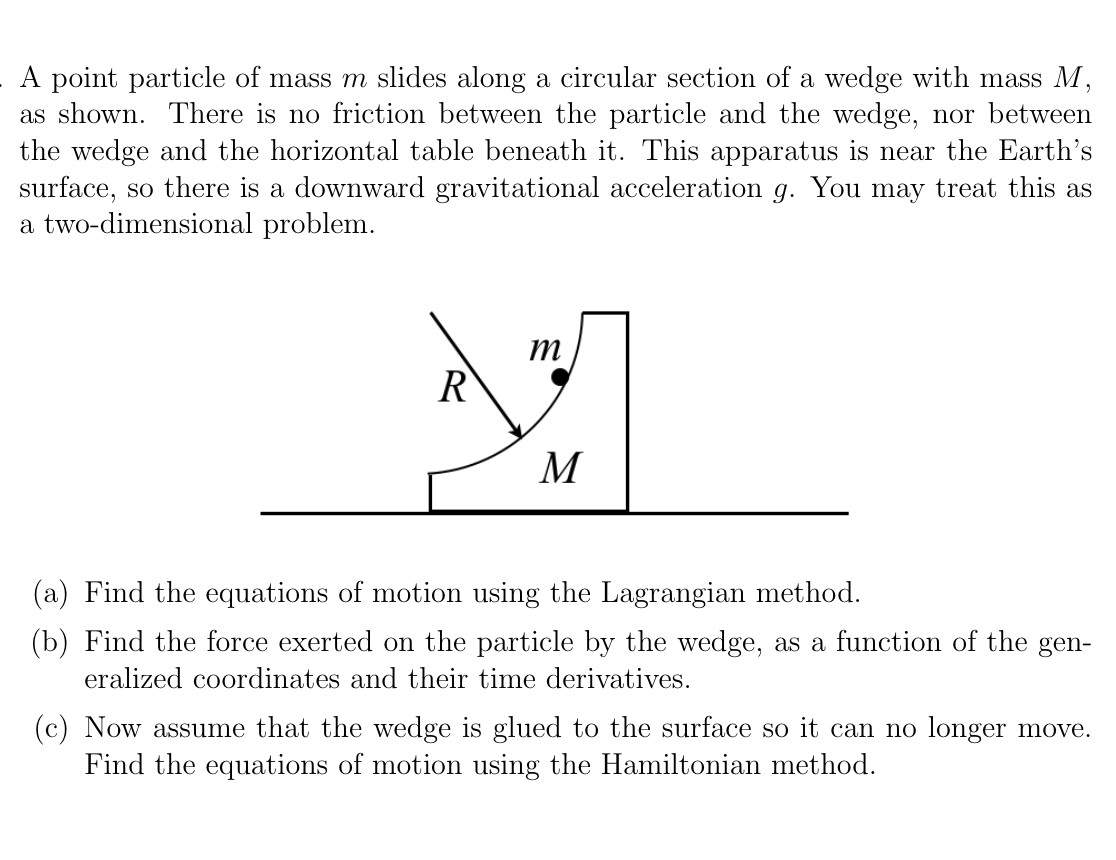# A point particle of mass m slides along a circular section of a wedge with mass M, as shown. There is no friction between the particle and the wedge, nor between the wedge and the horizontal table beneath it. This apparatus is near the Earth's surface, so there is a downward gravitational acceleration g. You may treat this as a two-dimensional problem. R M (a) Find the equations of motion using the Lagrangian method. (b) Find the force exerted on the particle by the wedge, as a function of the gen- eralized coordinates and their time derivatives. (c) Now assume that the wedge is glued to the surface so it can no longer move. Find the equations of motion using the Hamiltonian method.

Questionhelp_outlineImage TranscriptioncloseA point particle of mass m slides along a circular section of a wedge with mass M, as shown. There is no friction between the particle and the wedge, nor between the wedge and the horizontal table beneath it. This apparatus is near the Earth's surface, so there is a downward gravitational acceleration g. You may treat this as a two-dimensional problem. R M (a) Find the equations of motion using the Lagrangian method. (b) Find the force exerted on the particle by the wedge, as a function of the gen- eralized coordinates and their time derivatives. (c) Now assume that the wedge is glued to the surface so it can no longer move. Find the equations of motion using the Hamiltonian method. fullscreen

### Want to see this answer and more?

Experts are waiting 24/7 to provide step-by-step solutions in as fast as 30 minutes!*

*Response times may vary by subject and question complexity. Median response time is 34 minutes for paid subscribers and may be longer for promotional offers.
Tagged in
Science